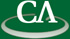Computing all Graphs on 11 vertices in Maple

Michael Monagan, CECM

Thursday May 18th, 2006 at 10:30am in K9509.

```Abstract:

I will describe an algorithm of Reid and Colburn from 1981 to generate
all graphs on n vertices and show some results for n=11 vertices using
a Maple implementation of the algorithm.  The main difficulty is space.
There are  15, 24, 37, 53, 70, 86, 99, 106, and 108 million different
graphs for 20, 21, 22, 23, 24, 25, 25, 27 and 28 edges, respectively.

I will sketch out the details of how to do this in Maple.
We can represent the graphs as 55 bit integers so that each graph, when
encoded as an integer, can be stored in one word on a 64 bit computer in
Maple.  We compute 60 bit integer hash codes for each one from their
characteristic polynomials to partition the large set of candidate graphs
into small sets.  Using a hash table in Maple to store the graphs,
indexed by these hash codes, this requires asymptotically 2 words
of storage per graph, hence, about 1.6 giga bytes of storage for
storing 100 million graphs.

```